# Clocks

How long path will walk the end of the clock's minute-hand long 7 cm for 330 minutes? How long will walk in the same time hour-hand?

Result

minute hand walk:  241.9 cm
minute hand walk:  20.2 cm

#### Solution:Leave us a comment of example and its solution (i.e. if it is still somewhat unclear...):Be the first to comment!#### To solve this example are needed these knowledge from mathematics:

Do you want to convert length units?

## Next similar examples:

1. ClocksHow long track travel the second hand in 46 hours, the end of which is 4 cm long.
2. Obtuse angleWhich obtuse angle is creating clocks at 17:00?
3. Minute-hand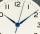How long distance will travel a large (minute) hand on the clock for 47 minutes, if its length is 43 cm?
4. Wheel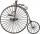Diameter of motocycle wheel is 52 cm. How many times rotates wheel on roand long 2 km?
5. Little hand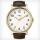What angle shifted little hand on the clock after one hour and 38 minutes?
6. MineWheel in traction tower has a diameter 5 m. How many meters will perform an elevator cabin if wheel rotates in the same direction 49 times?
7. Saw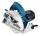Blade circular saw with a diameter 42 cm turns 825 times per minute. Expresses his cutting speed in meters per minute.
8. Freight and passenger car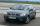The truck starts at 8 pm at 30 km/h. Passenger car starts at 8 pm at 40 km/h. Passenger arrives in the destination city 1 hour and 45 min earlier. What is the distance between the city of departure and destination city?
9. Two gears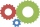The two gears fit together. The larger gear has 32 teeth, the smaller has 20 teeth less. How many times does turn a smaller gear if the bigger gear turns three times?
10. Circle r,DCalculate the diameter and radius of the circle if it has length 52.45 cm.
11. CircleWhat is the radius of the circle whose perimeter is 6 cm?
12. FlowerbedIn the park there is a large circular flowerbed with a diameter of 12 m. Jakub circulated him ten times and the smaller Vojtoseven times. How many meters each went by and how many meters did Jakub run more than Vojta?
13. Ferris wheel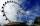Ferris wheel reaches to 22 m tall and moves at the speed of 0.5m/s. During one drive wheel rotates three times. What is the total drive time?
14. Wheel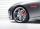How many times turns the wheel of a passenger car in one second if car run at speed 100 km/h. Wheel diameter is d = 62 cm.Determine the radius of the circle, if its perimeter and area is the same number.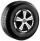What is the wheel diameter if on the 0.38 km track turns 128 times?Base of building is circle with diameter 25 m. Calculate the circumference of a circular trench witch diameter is 41 cm wider than the diameter of the base.